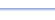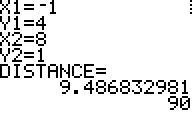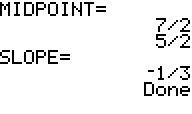Program: DISTANCE

DISTANCE

• Finds the distance between 2 points on the (x , y) coordinate plane (free version).
• Finds the midpoint of a segment, given the coordinates of the endpoints.
• Finds the slope of a line, given two points on the line.

Useful for:

• SAT
• ACT
• Algebra 1 & 2
• Geometry
• Trigonometry
• Precalculus
• Calculus

See full list of programs

Try these problems using DISTANCE:
on MathPro Q&A
in SAT/ACT books(Free version finds distance only.)
(free version available here)
interpret as the square root of 90 --->
------>
Example

Q: Find the length, midpoint, and slope of the segment joining points (-1, 4) and (8,1).

Solution: Run DISTANCE.  How?

Enter the values
for x1, y1, x2, y2
as shown:

The program reports
the following distance
information:

Press ENTER for more.
The program reports
the x- and y- values of
the midpoint.

Press ENTER for more.
The program reports
the slope.

Interpretation:
The length is about 9.4, or 90,
which is 310  (use RADICALN to
simplify).
The midpoint is (7/2 , 5/2).
The slope is -1/3.